# roipoly

Specify polygonal region of interest (ROI)

## Syntax

``BW = roipoly``
``BW = roipoly(I)``
``BW = roipoly(I,xi,yi)``
``BW = roipoly(x,y,I,xi,yi)``
``[BW,xi2,yi2] = roipoly(___)``
``[x2,y2,BW,xi2,yi2] = roipoly(___)``
``roipoly(___)``

## Description

````BW = roipoly` creates an interactive polygon tool associated with the image displayed in the current figure. With this syntax and the other interactive syntaxes, the polygon selection tool blocks the MATLAB® command line until you complete the operation. `roipoly` returns the mask as a binary image, setting pixels inside the ROI to `1` and pixels outside the ROI to `0`.For more information about using the polygon selection tool, see Interactive Behavior.```
````BW = roipoly(I)` displays the grayscale or RGB image `I` in a figure window and creates an interactive polygon selection tool associated with the image.```

example

````BW = roipoly(I,xi,yi)` specifies the polygon vertices as X-Y coordinates `xi` and `yi` in the default spatial coordinate system.```
````BW = roipoly(x,y,I,xi,yi)` defines a nondefault spatial coordinate system using the vectors `x` and `y`. The polygon vertices have coordinates `xi` and `yi` in this coordinate system.```
````[BW,xi2,yi2] = roipoly(___)` also returns the coordinates of the vertices of the closed polygon, `xi2` and `yi2`.```
````[x2,y2,BW,xi2,yi2] = roipoly(___)` also returns the image limits in `x2` and `y2`.```
````roipoly(___)` without an output argument displays the resulting mask image in a new figure window.```

## Examples

collapse all

Read an image into the workspace.

`I = imread('eight.tif');`

Define the vertices of the mask polygon.

```c = [222 272 300 270 221 194]; r = [21 21 75 121 121 75];```

`BW = roipoly(I,c,r);`

Display the original image and the polygonal mask.

`imshow(I)`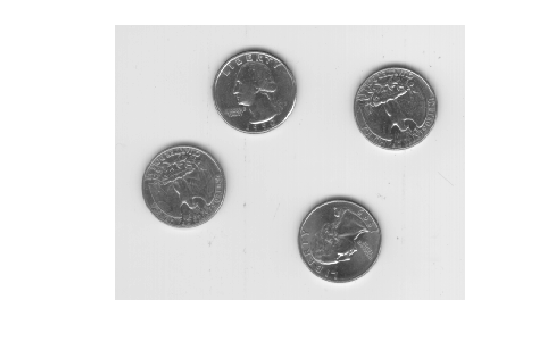```figure imshow(BW)```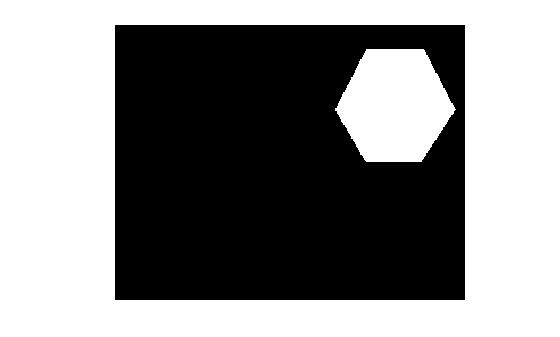## Input Arguments

collapse all

Grayscale or RGB image, specified as an m-by-n numeric matrix for a grayscale image, or an m-by-n-by-3 numeric array for an RGB image.

x- or y-coordinate of polygon vertices, specified as numeric vectors of equal length. If you specify a nondefault coordinate system using the `x` and `y` arguments, then `xi` and `yi` specify coordinates in this coordinate system. Otherwise, `xi` and `yi` specify coordinates in the default coordinate system.

Image extent in world X-Y coordinates, specified as 2-element numeric vectors of the form `[min max]`. The values of `x` and `y` set the image `XData` and `YData`.

## Output Arguments

collapse all

Binary image, returned as an m-by-n logical matrix.

Data Types: `logical`

x- or y-coordinate of vertices of the closed polygon, returned as numeric vectors of equal length. The first and last element in each vector are identical, so that the polygon is closed. If you specify a nondefault coordinate system using the `x` and `y` arguments, then `xi2` and `yi2` are this coordinate system. Otherwise, `xi2` and `yi2` are in the default coordinate system.

### Note

`roipoly` always produces a closed polygon. If you specify input vertex positions of a closed polygon (such that the last pair of coordinates is identical to the first pair), then the length of the output coordinate vectors is equal to the number of points specified. If the points specified do not describe a closed polygon, then `roipoly` adds a final point having the same coordinates as the first point. In this case the length of the output coordinate vectors is one greater than the number of points specified.

Image extent in world X-Y coordinates, returned as 2-element numeric vectors of the form `[min max]`. If you specify a nondefault coordinate system using the `x` and `y` arguments, then `x2` and `y2` are equal to these values. Otherwise, `x2` and `y2` are equal to the original image `XData` and `YData`.

collapse all

### Interactive Behavior

The polygon selection tool enables you to select and adjust polygon vertices interactively using the mouse.

When the polygon tool is active, the pointer changes to cross hairs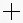when you move the pointer over the image in the figure. Using the mouse, you specify the region by selecting vertices of the polygon. You can move or resize the polygon using the mouse. When you are finished positioning and sizing the polygon, create the mask by double-clicking, or by right-clicking inside the region and selecting Create mask from the context menu.

The figure illustrates a polygon defined by multiple vertices. The following table describes all the interactive behavior of the polygon tool.Interactive BehaviorDescription
Closing the polygon. (Completing the region-of-interest.)

Use any of the following mechanisms:

• Move the pointer over the initial vertex of the polygon that you selected. The pointer changes to a circle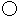. Click either mouse button.

• Double-click the left mouse button. This action creates a vertex at the point under the mouse pointer and draws a straight line connecting this vertex with the initial vertex.

• Right-click the mouse. This draws a line connecting the last vertex selected with the initial vertex; it does not create a new vertex at the point under the mouse.

Moving the entire polygonMove the pointer inside the region. The pointer changes to a fleur shape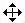. Click and drag the polygon over the image.
Deleting the polygon

Press Backspace, Escape or Delete, or right-click inside the region and select Cancel from the context menu.

Note: If you delete the ROI, the function returns empty values.

Moving a vertex. (Reshaping the region-of-interest.)Move the pointer over a vertex. The pointer changes to a circle. Click and drag the vertex to its new position.
Adding a new vertex.Move the pointer over an edge of the polygon and press the A key. The pointer changes shape to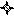. Click the left mouse button to create a new vertex at that point on the edge.
Deleting a vertex. (Reshaping the region-of-interest.)Move the pointer over the vertex. The pointer changes to a circle. Right-click and select Delete vertex from the context menu. `roipoly` draws a new straight line between the two vertices that were neighbors of the deleted vertex.
Changing the color of the polygonMove the pointer anywhere inside the boundary of the region and click the right mouse button. Select Set color from the context menu.
Retrieving the coordinates of the vertices Move the pointer inside the region. Right-click and select Copy position from the context menu to copy the current position to the Clipboard. The position is an n-by-2 array containing the x- and y-coordinates of each vertex, where n is the number of vertices.

## Tips

• For any of the `roipoly` syntaxes, you can replace the input image `I` with two arguments, `m` and `n`, that specify the row and column dimensions of an arbitrary image. For example, these commands create a 100-by-200 binary mask.

```c = [112 112 79 79]; r = [37 66 66 37]; BW = roipoly(100,200,c,r); ```

If you specify `m` and `n` with an interactive form of `roipoly`, an `m`-by-`n` black image is displayed. Use the mouse to specify a polygon within this image.